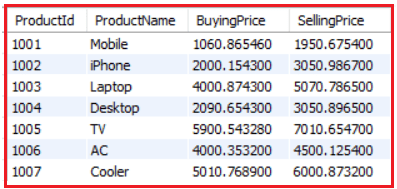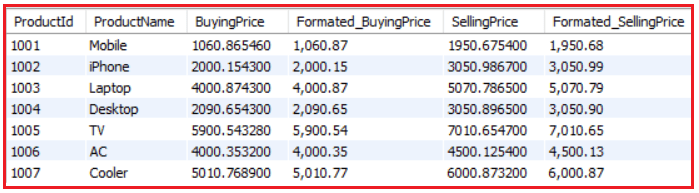# MySQL FORMAT Function

## MySQL FORMAT Function with Examples

In this article, I am going to discuss MySQL FORMAT Function with Examples. Please read our previous article where we discussed MySQL ROUND Function with Examples.

##### MySQL FORMAT Function

Similar to the ROUND function, The FORMAT function is also used to round a floating-point number to the given decimal numbers. The difference is that, the MySQL FORMAT() function formats the number to a format like “#,###,###.##”, rounded to a specified number of decimal places, and then it returns the result as a string. The FORMAT function also only works on numeric columns.

##### MySQL FORMAT Function Syntax:

Following is the syntax to use the FORMAT function.

FORMAT(number, decimals, [locale])

###### Parameters:

The FORMAT function accepts two parameters as follows:

1. number: Required. The number to be rounded
2. decimals: Required. The Number of decimal places up to which the given number is to be rounded. If it is negative, then the number is rounded to the left side of the decimal point. If this parameter is 0, this function returns a string with no decimal places.
3. Locale: Optional. It specifies the locale to use that determines the decimal point result, thousands of separators, and grouping between separators. By default, MySQL will use an en_us locale.

Returns: The MySQL FORMAT function formats the given number, round off it to a certain decimal place, and return the number in the form of a string.

###### Example: Formatting off a number with Decimal is positive.

Positive Number:
SELECT FORMAT(250500.5634) AS Formatted_Number;
Output: 250,500.56

Negative Number:
SELECT FORMAT(-250500.5634, 2) AS Formatted_Number;
Output: -250,500.56

###### Example: Formatting off a number when the decimal is negative.

Formatting a Positive number up to 2 decimal places
SELECT FORMAT (22516.67519, -2) AS Formatted_Number;
Output: 22,517

Formatting a Negative number up to 3 decimal places
SELECT FORMAT (-22516.67519, -3) AS Formatted_Number;
Output: -22,517

###### Example: Formatting off a number to 0 decimal places.

Formatting a Positive number up to 0 decimal places
SELECT FORMAT (22516.67519, 0) AS Formatted_Number;
Output: 22,517

Formatting a Negative number up to 0 decimal places
SELECT FORMAT (-22516.67519, 0) AS Formatted_Number;
Output: -22,517

##### Example: Using locale.

As of now, the examples we discussed uses en_US locale by default. This is because we have not provided any locale with the MySQL FORMAT function. Now, the following example going to use de_DE locale instead of en_US.

SELECT FORMAT (22516.67519, 2, ‘de_DE’) AS Formatted_Number;
Output: 22.516,68

##### Working with database table:

The MySQL FORMAT Function can also be used to format the decimal values and return them as a string for the database table column data. To understand this concept, we are going to use the following Product table.Please use the below SQL Script to create and populate the Product table with the required sample data.

```CREATE TABLE Product(
ProductId INT PRIMARY KEY,
ProductName VARCHAR(100) NOT NULL,
SellingPrice DECIMAL(13, 6) NOT NULL
);

INSERT INTO Product VALUES (1001, 'Mobile', 1060.865460, 1950.675400);
INSERT INTO Product VALUES (1002, 'iPhone', 2000.154300, 3050.986700);
INSERT INTO Product VALUES (1003, 'Laptop', 4000.874300, 5070.786500);
INSERT INTO Product VALUES (1004, 'Desktop', 2090.654300, 3050.896500);
INSERT INTO Product VALUES (1005, 'TV', 5900.543280, 7010.654700);
INSERT INTO Product VALUES (1006, 'AC', 4000.353200, 4500.125400);
INSERT INTO Product VALUES (1007, 'Cooler', 5010.768900, 6000.873200);
```

Now, we are going to find the formatted values for the Price column of the Product table up to 2 decimal points by executing the below SQL script.

```SELECT ProductId, ProductName,
SellingPrice,
FORMAT(SellingPrice, 2) Formated_SellingPrice
FROM Product;
```

When you execute the above script, you will get the following output.1.